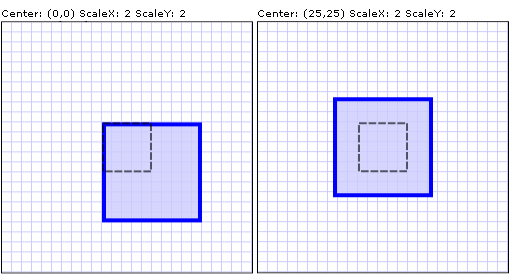# How to: Scale an Element

This example shows how to use a ScaleTransform to scale an element.

Use the ScaleX and ScaleY properties to resize the element by the factor you specify. For example, a ScaleX value of 1.5 stretches the element to 150 percent of its original width. A ScaleY value of 0.5 shrinks the height of an element by 50 percent.

Use the CenterX and CenterY properties to specify the point that is the center of the scale operation. By default, a ScaleTransform is centered at the point (0,0), which corresponds to the upper-left corner of the rectangle. This has the effect of moving the element and also of making it appear larger, because when you apply a Transform, you change the coordinate space in which the object resides.

The following example uses a ScaleTransform to double the size of a 50-by-50 Rectangle. The ScaleTransform has a value of 0 (the default) for both CenterX and CenterY.

## Example

``````<!-- Scales a rectangle by 200% from a center of (0,0).-->
<Rectangle Height="50" Width="50" Fill="#CCCCCCFF"
Stroke="Blue" StrokeThickness="2"
Canvas.Left="100" Canvas.Top="100">
<Rectangle.RenderTransform>
<ScaleTransform CenterX="0" CenterY="0" ScaleX="2" ScaleY="2" />
</Rectangle.RenderTransform>
</Rectangle>
``````

Typically, you set CenterX and CenterY to the center of the object that is scaled: (Width/2, Height/2).

The following example shows another Rectangle that is doubled in size; however, this ScaleTransform has a value of 25 for both CenterX and CenterY, which corresponds to the center of the rectangle.

``````<!-- Scales a rectangle by 200% from a center of (25,25).-->
<Rectangle Height="50" Width="50" Fill="#CCCCCCFF"
Canvas.Left="100" Canvas.Top="100" Stroke="Blue" StrokeThickness="2">
<Rectangle.RenderTransform>
<ScaleTransform CenterX="25" CenterY="25" ScaleX="2" ScaleY="2" />
</Rectangle.RenderTransform>
</Rectangle>
``````

The following illustration shows the difference between the two ScaleTransform operations. The dotted line shows the size and position of the rectangle before scaling.Two ScaleTransform operations with identical ScaleX and ScaleY values but different centers

For the complete sample, see 2D Transforms Sample.